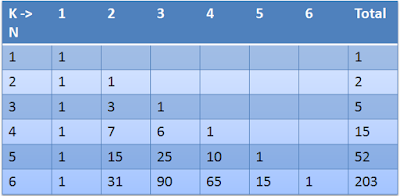# Permutations: Balls and boxes related problems

Problems related to balls and boxes can be classified mainly into 4 categories.

Balls – Distinct,  Boxes – Distinct
Balls-   Similar,    Boxes – Distinct
Balls -  Distinct,  Boxes – Similar
Balls – Similar,    Boxes - SimilarCase 1: Balls – Distinct, Boxes – Distinct

Condition 1: A box can take any Number of balls
We know that if a box can take any number of balls let us take a ball No.9 which can go into any of the four distinct boxes.  Next ball can also go into any of these four boxes.  Similarly, remaining balls also can go into any of these 4 boxes. So total number of ways are 4 x 4 x 4 x 4 = 256
Formula : ${(boxes)^{balls}}$

Condition 2: A box can take minimum one ball: (Assume there are 5 balls and 3 boxes)

This case is one of the most interesting case. This can be solved in many ways.

Method 1:

We first divide 5 balls into 3 different groups and then we allocate these groups into 3 boxes.
We divide 5 balls into 3 groups in two ways: 2, 2, 1 or 3, 3, 1
Now (m+n+p) objects may be divided into 3 groups containing m, n, p objects is given by $\displaystyle\frac{{(m + n + p)!}}{{m! \times n! \times p!}}$
But when any of m,n, or p are same we have to divide by that similar numbers. Here in 2, 2, 1 two 2's are same. So final answer should be divided by 2!.
$\displaystyle\frac{{(5)!}}{{2! \times 2! \times 1!}} \times \displaystyle\frac{1}{{2!}}$ = 15 ways
Similarly 5 balls may be divided into 3, 1, 1 are $\displaystyle\frac{{(5)!}}{{3! \times 1! \times 1!}} \times \displaystyle\frac{1}{{2!}}$ = 10 ways (Here 1, 1 are same)
Now each of these divisions are put into 3 boxes in 3! ways. So total ways are 3! x ( 15 + 10) = (3! x 25) = 150

Method 2:

Division of 5 balls into 3 groups of 2, 2, 1 can be done like this $^5{C_2}{ \times ^3}{C_2}{ \times ^1}{C_2} \times \displaystyle\frac{1}{{2!}}$ = 15
Division of 5 balls into 3 groups of 3, 1, 1 can be done like this $^5{C_3}{ \times ^2}{C_1}{ \times ^1}{C_1} \times \displaystyle\frac{1}{{2!}}$ = 10
Total divisios are 25 and total distributions are 25 x 3! = 150

Method 3:

Distribution of 5 distinct objects into 3 groups is equivalent to total onto mapping from a set of 5 to a set of 3 and which can be calculated by the formula :
${r^n} - {}^r{C_1}.{(r - 1)^n} + {}^r{C_2}.{(r - 2)^n} + {}^r{C_3}.{(r - 3)^n} - ......$
So ${3^5} - {}^3{C_1}.{(3 - 1)^5} + {}^3{C_2}.{(3 - 2)^5}$ = 150

Case 2: Balls – Similar, Boxes – Distinct

Suppose your dad has given you 100 rupees to buy ice-creasms.  Let us say an ice cream costs you Rs.25.  In an ice cream shop there are 5 varieties of ice cream available. Chololate, Vanilla, strawberry, Butter scotch, Pista.You can buy any varieties but you can buy maximum 4 ice creams as an ice cream costs you Rs.25 each.
So number of ice creams you are going to buy is
Chololate +Vanilla + Strawberry + Butter scotch + Pista = 4
This is a problem of finding intezer solutions to the above equation where sum of all the numbers in the places of 5 different ice creams is equal to 4.
We can apply the same logic to the balls and boxes where balls are similar (as ice creams of same variety are similar) but the sum of the balls in all the boxes together must equal to 4.

Condition 1: A box can take any Number of balls
Now A + B + C + D = 4 where any of these numbers can be Zero.
Number of intezer solutions of these equation where 0 is allowed is = $\left( {n + k - 1} \right){C_{k - 1}}$ (here k= number of boxes, n= number of balls)
$\Rightarrow \left( {4 + 4 - 1} \right){C_{4 - 1}} \Rightarrow 7{C_3}$

Condition 2: A box can take minimum 1 Ball
In this case we should take only natural numbers as 0 is not allowed.
Number of intezer solutions where 0 is not allowed is = $\left( {n - 1} \right){C_{k - 1}}$ = $\Rightarrow \left( {4 - 1} \right){C_{4 - 1}} \Rightarrow 3{C_3} = 1$

Case 3: Balls – Distinct, Boxes – Similar
This is one of the typical probelm where we attempt to solve it by using a special recurrence table called Stirling numbers of second kind.Condition 1: A box can take any number of balls
Here column N represents number of balls and K represents number of boxes.
Now 4 ball and 4 boxes can be arranged in 1 + 7 + 6 + 1 = 15 ways.

Condition 2: A box can take minimum one balls
Now we should take the intersection point of n = 4 and k= 4 so answer = 1

Case 4: Balls – Similar, Boxes – Similar
We use another recurrence table to solve this question

Condition 1: A box can take any number of balls
Add all the numbers in the 4th row and 4th column so answer = 5

Condition 2: A box can take minimum one balls
Here take the intersection of 4th row and 4th column = 1